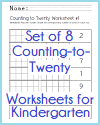Grade 1: Number and Operations in Base Ten
 Share the learning joy!

Grade 1 Math: Number and Operations in Base Ten
 www.studenthandouts.com > First Grade > First Grade Math > Number and Operations in Base Ten

CCSS.MATH.CONTENT.1.NBT.A.1 - Count to 120, starting at any number less than 120. In this range, read and write numerals and represent a number of objects with a written numeral.

CCSS.MATH.CONTENT.1.NBT.B.2 - Understand that the two digits of a two-digit number represent amounts of tens and ones. Understand the following as special cases:

CCSS.MATH.CONTENT.1.NBT.B.2.A - 10 can be thought of as a bundle of ten ones — called a "ten."

CCSS.MATH.CONTENT.1.NBT.B.2.B - The numbers from 11 to 19 are composed of a ten and one, two, three, four, five, six, seven, eight, or nine ones.Shade Boxes to 20; Set of 8 Worksheets
CCSS.MATH.CONTENT.1.NBT.B.2.C - The numbers 10, 20, 30, 40, 50, 60, 70, 80, 90 refer to one, two, three, four, five, six, seven, eight, or nine tens (and 0 ones).

CCSS.MATH.CONTENT.1.NBT.B.3 - Compare two two-digit numbers based on meanings of the tens and ones digits, recording the results of comparisons with the symbols >, =, and <.

CCSS.MATH.CONTENT.1.NBT.C.4 - Add within 100, including adding a two-digit number and a one-digit number, and adding a two-digit number and a multiple of 10, using concrete models or drawings and strategies based on place value, properties of operations, and/or the relationship between addition and subtraction; relate the strategy to a written method and explain the reasoning used. Understand that in adding two-digit numbers, one adds tens and tens, ones and ones; and sometimes it is necessary to compose a ten.

CCSS.MATH.CONTENT.1.NBT.C.5 - Given a two-digit number, mentally find 10 more or 10 less than the number, without having to count; explain the reasoning used.

CCSS.MATH.CONTENT.1.NBT.C.6 - Subtract multiples of 10 in the range 10-90 from multiples of 10 in the range 10-90 (positive or zero differences), using concrete models or drawings and strategies based on place value, properties of operations, and/or the relationship between addition and subtraction; relate the strategy to a written method and explain the reasoning used.